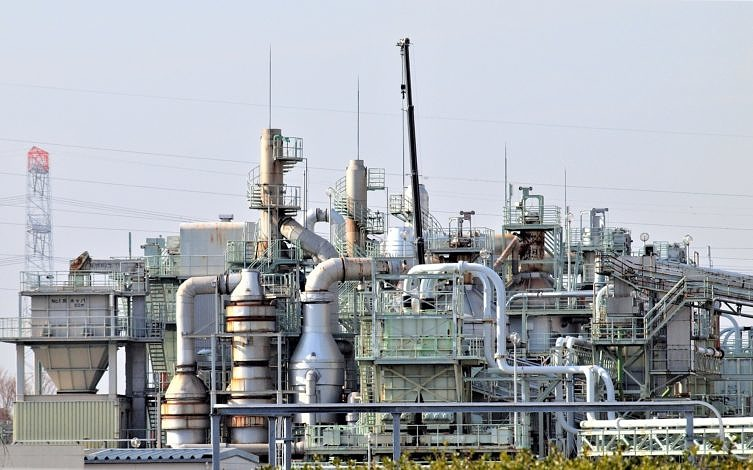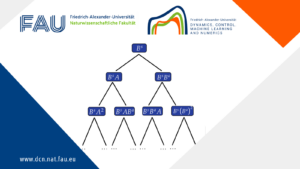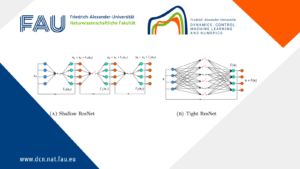# Gas networks uncertainty and Probust constraints: model, distribution and optimization## Gas networks uncertainty and Probust constraints: model, distribution and optimization

Gas transport and distribution systems are usually operating under complex pipelines network topologies which make possible gas flow over interconnected stations -nodes- and branches under a variety of conditions, especially large-scale gas infrastructures. As many applications contain different types of uncertainties (i.e. roughness coefficients of the pipe) that require robust as well as probabilistic treatments, we deal with a class of joint probabilistic/robust constraints: probust constraints.

Gas network uncertainty
Illustrating the application of this new class of probust constraints to optimization problems in gas transport under uncertainty in the exit and entry loads, we focus on complex uncertain gas network optimization problems:

• we show the relevance of these problems to maximize free booked capacities in an algebraic model for a stationary gas network
• we present approaches for finding their solutions
• we study the problem of controlling a transient system governed by the wave equation

Modeling rational decisions with optimization problems often means random variables occur in the constraints. To deal with uncertainty in the constraints of an optimization problem there are two approaches:

1. Probabilistic constraints
2. Robust optimization

In both, nature and source of uncertainty are needed on the decision support schemes with non-deterministic parameters while the objective and constraints of the problem are balanced.

Probust constraints

Considering the typical form for an optimization problem dealing with uncertain variables:
min_x \hspace{2cm} g_o(x) \\ \text{subject to} \hspace{1.3cm} g_1(x,z) \geq 0 (i=1,...,k) \hspace{2cm} (1)

with x \in R_n as a decision vector, z \in R_m a an uncertain parameter, g_0:R_n \rightarrow R as the objective function and g:R_n \times R_m \rightarrow R_k as the constraint mapping.

1- Probabilistic constraints. Often developed in case the uncertainties (chance constraints) can be assumed to follow a certain probability distribution, this approach is based on the assumption that historical data is available to estimate the probability distribution of the uncertain parameter and thus considering it as a random vector ξ taking values in R_m . Then (1) may be given the form:
min_x \hspace{2cm} g_0(x) \\ \text{subject to} \hspace{1.3cm} \mathbb{P}(g(x,\varepsilon) \geq 0) \geq p \in (0,1] \hspace{1.7cm} (2)

Here, a decision x is declared to be feasible if and only if the original random inequality system (1) is satisfied with a prescribed probability p, a level usually chosen close to, but not identical to one: higher values of p lead to a smaller set of feasible decisions x in (2), hence to optimal solutions at higher costs. Concerning the random variable ξ we essentially focus on continuous ones.

2- Robust optimization. It’s an alternative approach usually applied in case solutions are sought that are feasible for all realizations of uncertainties within some predefined uncertainty set. It applies when the uncertain parameter u is completely unknown or non-stochastic due to a lack of available data. Then, satisfaction of the uncertain inequality system (1) is required for every realization of the uncertainty parameter within some uncertainty set U ⊆ R^m containing infinitely many elements in general, so that one arrives at the following optimization problem:
min_x \hspace{2cm} g_0(x) \\ \text{subject to} \hspace{1.3cm} g(x,u) \geq 0 \hspace{0.3cm} \forall u \in U \hspace{2.5cm} (3)

We notice that both optimization models with probabilistic and robust constraints are deterministic reformulations of (1), since they depend only on the decision vector x but no longer on the outcome of the uncertain parameter z. A central issue in robust optimization is the appropriate choice of the uncertainty set U.

Probabilistic programming and robust optimization commonly dealt separately, led us to consider uncertain inequalities (2) in which the uncertain variable has a stochastic and a non-stochastic part, i.e., z = (ξ, u) into a typical example is optimization of gas transport in the presence of uncertain loads that faces uncertain variables of both:

• Historical data are available (stochastic uncertainty) for the exit loads in many situations
• Observations can hardly be accessed (non-stochastic uncertainty) for entry loads, or for example, uncertain roughness coefficients in pipes

Formulating a probabilistic constraint (w.r.t. ξ ) involving a robustified (w.r.t. u) infinite inequality system:
P (g(x, ξ, u) ≥ 0 ∀u ∈ U) ≥ p \hspace{4cm} (4)

These are probust constraints recently investigated as hybrid robust/chance-constraints in  and as probabilistic/robust constraints in .

We note that even the more complex situation of the uncertainty set depending on the decision and random variable plays an increasing role in applications. Here, the constraint becomes
P (g(x, ξ, u) ≥ 0 ∀u ∈ U(x, ξ )) ≥ p \hspace{3.3cm} (5)

where the inner part resembles constraint sets arising in generalized semi-infinite optimization .

We note that yet another form of combining the probabilistic and robust parts of the inequality system would result from interchanging their arrangements in (4):
P (g(x, ξ, u) ≥ 0) ≥ p ∀u ∈ U

The probust constraints provide a tool that allows to treat optimization problems where both types of data occur simultaneously.

See more details at  and .

References

 Gugat M., Mateos, M. & Tröltzsch, F. Exponential Stability for the Schlögl System by Pyragas Feedback. Vietnam J. Math. 48, 769–790 (2020). https://doi.org/10.1007/s10013-020-00382-7

 Adelhütte D., Aßmann D., Grandón T.G., Gugat M., et al. Joint Model of Probabilistic-Robust (Probust) Constraints Applied to Gas Network Optimization. Vietnam J. Math. (2020). https://doi.org/10.1007/s10013-020-00434-y

 Grandón, T.G., Heitsch, H., Henrion, R.: A joint model of probabilistic/robust constraints for gas transport management in stationary networks. Comput. Manag. Sci. 14, 443–460 (2017)

 van Ackooij, W., Frangioni, A., de Oliveira, W. Inexact stabilized Benders’ decomposition approaches with application to chance-constrained problems with finite support. Comput. Optim. Appl. 65, 637–669 (2016)

 Vázquez, F.G., Rückmann, J.-J., Stein, O., Still, G. Generalized semi-infinite programming: a tutorial. J. Comput. Appl. Math. 217, 394–419 (2008)

### You might like!#### FAU MoD Lecture: Free boundary regularity for the obstacle problem#### Stability of hyperbolic systems with non-symmetric partial dissipation#### PottsMGNet: A Mathematical Explanation of Encoder-Decoder Based Neural Networks